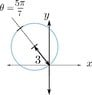# Line perpendicular to the ray

• Poetria

## Homework Statement

[/B]
Consider the line whose shortest distance to the origin is 5 and that is perpendicular to the ray ##\theta= \frac {5*\pi}{7}## for r>0
Find its polar equation ##r=r(\theta)## and ##\theta_1<\theta_2## in the interval ##[0, 2\pi]## such that ##r(\theta)\geq 0## for all ##\theta_1\leq\theta\leq\theta_2## as ##\theta## increases from ##\theta_1## to ##\theta_2##, the point ##(r(\theta), \theta)## traces the entire line once.

2. The attempt at a solution

m*x+b=y
##m=\tan(3/14*\pi)##
b=5/cos(2/7*pi)

$$-\frac{\frac 5 {\cos(\frac 2 7*\pi)}}{(\tan( \frac 3 {14}*\pi)*\cos(\theta)-\sin(\theta))}$$

#### Attachments

•images_polar_circlerotated.jpg
15 KB · Views: 438
Last edited by a moderator:

You have calculated b incorrectly. If you correct this, then your final answer will be right - but it's a long way round and a complicated expression. Try doing it without going through y = mx + b. Draw the ray, the line, and a point (r,θ) on the line. Can you derive a simpler expression for r(θ)?

•Poetria
You have calculated b incorrectly. If you correct this, then your final answer will be right - but it's a long way round and a complicated expression. Try doing it without going through y = mx + b. Draw the ray, the line, and a point (r,θ) on the line. Can you derive a simpler expression for r(θ)?

Something like:

##r=5*sec(\theta-\frac 5 7*\pi)##

That's what I got.

•Poetria
That's what I got.

Great. :) Many thanks. :)

Great. :) Many thanks. :)
Did you find ##\ \theta _1\ ## and ##\ \theta _2\ ## ?

•Poetria
Did you find ##\ \theta _1\ ## and ##\ \theta _2\ ## ?

Yes, this was easy:
$$\theta_1=\frac 3 {14}\pi$$
$$\theta_2=\frac {17}{14}\pi$$